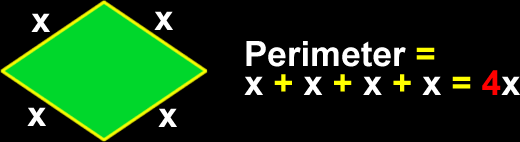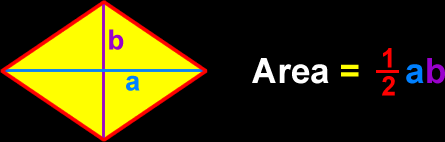# The Properties of a Rhombus

Definitions and formulas for the perimeter of a rhombus, the area of a rhombus,  properties of the angles and sides of a rhombus
Just scroll down or click on what you want and I'll scroll down for you!

 perimeter of a rhombus area of a rhombus sides and anglesof a rhombus properties of thediagonals of a rhombus properties of theangles of a rhombus

 The perimeter of a rhombus: To find the perimeter of a rhombus, just add up all the lengths of the sides:The area of a rhombus: To find the area of a rhombus, multiply the lengths of the two diagonals and divide by 2 (same as multiplying by 1/2):The sides and angles of a rhombus:The sides of a rhombus are all congruent (the same length.) Opposite angles of a rhombus are congruent (the same size and measure.)